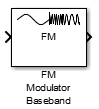Main Content

# FM Modulator Baseband

Modulate using FM method

• Library:
• Communications Toolbox / Modulation / Analog Baseband Modulation

•## Description

The FM Modulator Baseband block applies frequency modulation to a real input signal and returns a complex output signal.

## Ports

### Input

expand all

Input signal, specified as a real scalar, vector, or matrix.

Data Types: `double` | `single`

### Output

expand all

Output signal, returned as a real scalar, vector, or matrix. The data at this port has the same data type and size as the input signal.

Data Types: `double` | `single`

## Parameters

expand all

Frequency deviation of the modulator, in Hz, specified as a positive scalar. The system bandwidth is equal to twice the sum of the frequency deviation and the message bandwidth.

Type of simulation to run, specified as `Code generation` or `Interpreted execution`.

• `Code generation` –– Simulate the model by using generated C code. The first time you run a simulation, Simulink® generates C code for the block. The C code is reused for subsequent simulations unless the model changes. This option requires additional startup time, but the speed of the subsequent simulations is faster than `Interpreted execution`.

• `Interpreted execution` –– Simulate the model by using the MATLAB® interpreter. This option requires less startup time than the `Code generation` method, but the speed of subsequent simulations is slower. In this mode, you can debug the source code of the block.

## Block Characteristics

 Data Types `double` | `single` Multidimensional Signals `no` Variable-Size Signals `no`

## Algorithms

Represent a frequency modulated passband signal, Y(t), as

`$Y\left(t\right)=A\mathrm{cos}\left(2\pi {f}_{c}t+2\pi {f}_{\Delta }{\int }_{0}^{t}x\left(\tau \right)d\tau \right)\text{\hspace{0.17em}},$`

where A is the carrier amplitude, fc is the carrier frequency, x(τ) is the baseband input signal, and fΔ is the frequency deviation in Hz. The frequency deviation is the maximum shift from fc in one direction, assuming |x(t)| ≤ 1.

A baseband FM signal can be derived from the passband representation by downconverting it by fc such that

`$\begin{array}{c}{y}_{s}\left(t\right)=Y\left(t\right){e}^{-j2\pi {f}_{c}t}=\frac{A}{2}\left[{e}^{j\left(2\pi {f}_{c}t+2\pi {f}_{\Delta }{\int }_{0}^{t}x\left(\tau \right)d\tau \right)}+{e}^{-j\left(2\pi {f}_{c}t+2\pi {f}_{\Delta }{\int }_{0}^{t}x\left(\tau \right)d\tau \right)}\right]{e}^{-j2\pi {f}_{c}t}\\ =\frac{A}{2}\left[{e}^{j2\pi {f}_{\Delta }{\int }_{0}^{t}x\left(\tau \right)d\tau }+{e}^{-j4\pi {f}_{c}t-j2\pi {f}_{\Delta }{\int }_{0}^{t}x\left(\tau \right)d\tau }\right]\text{\hspace{0.17em}}.\end{array}$`

Removing the component at -2fc from ys(t) leaves the baseband signal representation, y(t), which is expressed as

`$y\left(t\right)=\frac{A}{2}{e}^{j2\pi {f}_{\Delta }{\int }_{0}^{t}x\left(\tau \right)d\tau }.$`

The expression for y(t) is rewritten as

`$y\left(t\right)=\frac{A}{2}{e}^{j\varphi \left(t\right)}\text{\hspace{0.17em}},$`

where $\varphi \left(t\right)=2\pi {f}_{\Delta }{\int }_{0}^{t}x\left(\tau \right)d\tau$, which implies that the input signal is a scaled version of the derivative of the phase, ϕ(t).

A baseband delay demodulator is used to recover the input signal from y(t).A delayed and conjugated copy of the received signal is subtracted from the signal itself,

`$w\left(t\right)=\frac{{A}^{2}}{4}{e}^{j\varphi \left(t\right)}{e}^{-j\varphi \left(t-T\right)}=\frac{{A}^{2}}{4}{e}^{j\left[\varphi \left(t\right)-\varphi \left(t-T\right)\right]}\text{\hspace{0.17em}},$`

where T is the sample period. In discrete terms, wn=w(nT), and

`$\begin{array}{l}{w}_{n}=\frac{{A}^{2}}{4}{e}^{j\left[{\varphi }_{n}-{\varphi }_{n-1}\right]}\text{\hspace{0.17em}}\text{,}\\ {v}_{n}={\varphi }_{n}-{\varphi }_{n-1}\text{\hspace{0.17em}}.\end{array}$`

The signal vn is the approximate derivative of ϕn, such that vnxn.

 Chakrabarti, I. H., and I, Hatai. “A New High-Performance Digital FM Modulator and Demodulator for Software-Defined Radio and Its FPGA Implementation.” International Journal of Reconfigurable Computing. Vol. 2011, No. 10.1155/2011, 2011, p. 10.

 Taub, Herbert, and Donald L. Schilling. Principles of Communication Systems. New York: McGraw-Hill, 1971, pp. 142–155.

## Support

#### Bridging Wireless Communications Design and Testing with MATLAB

Download white paper